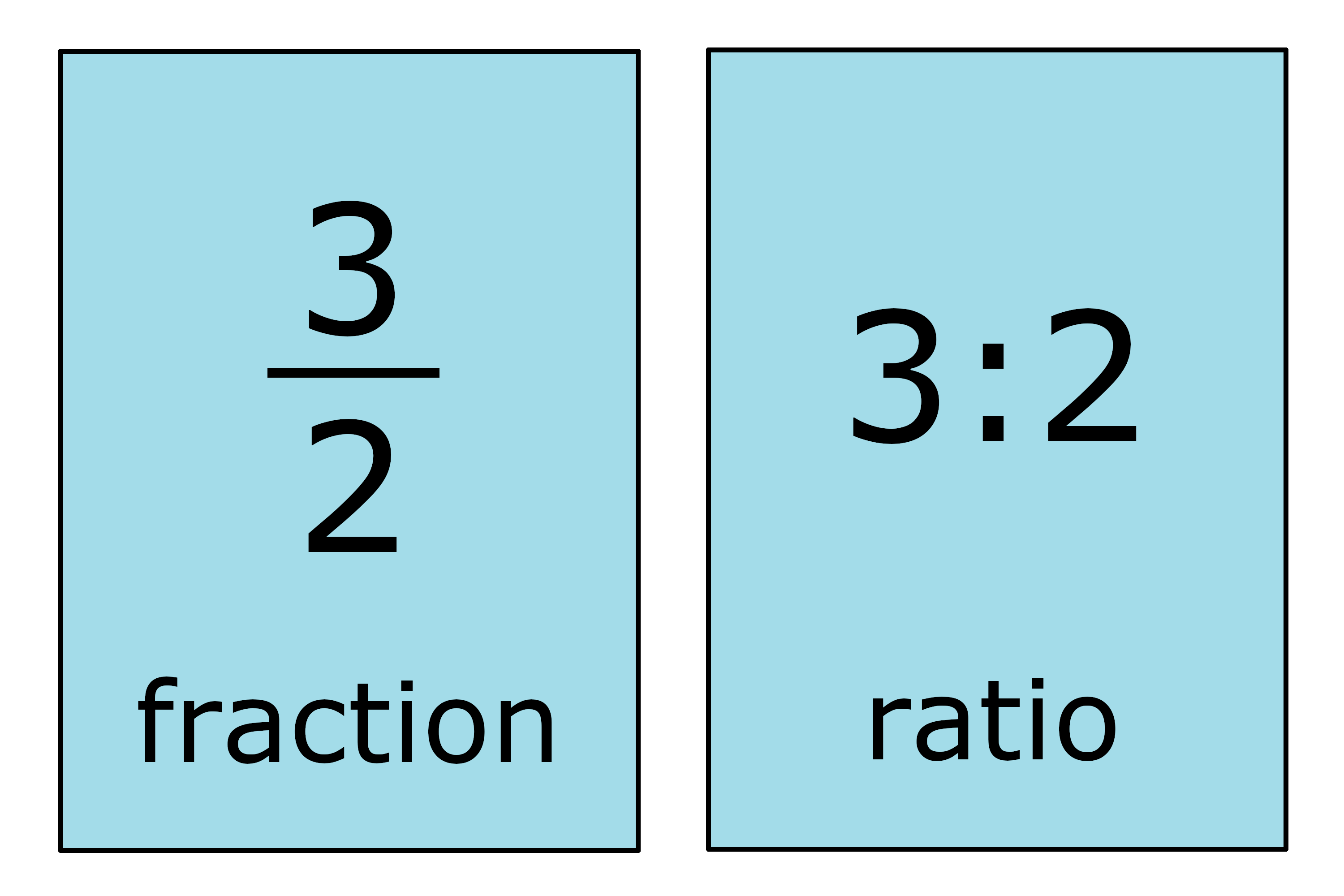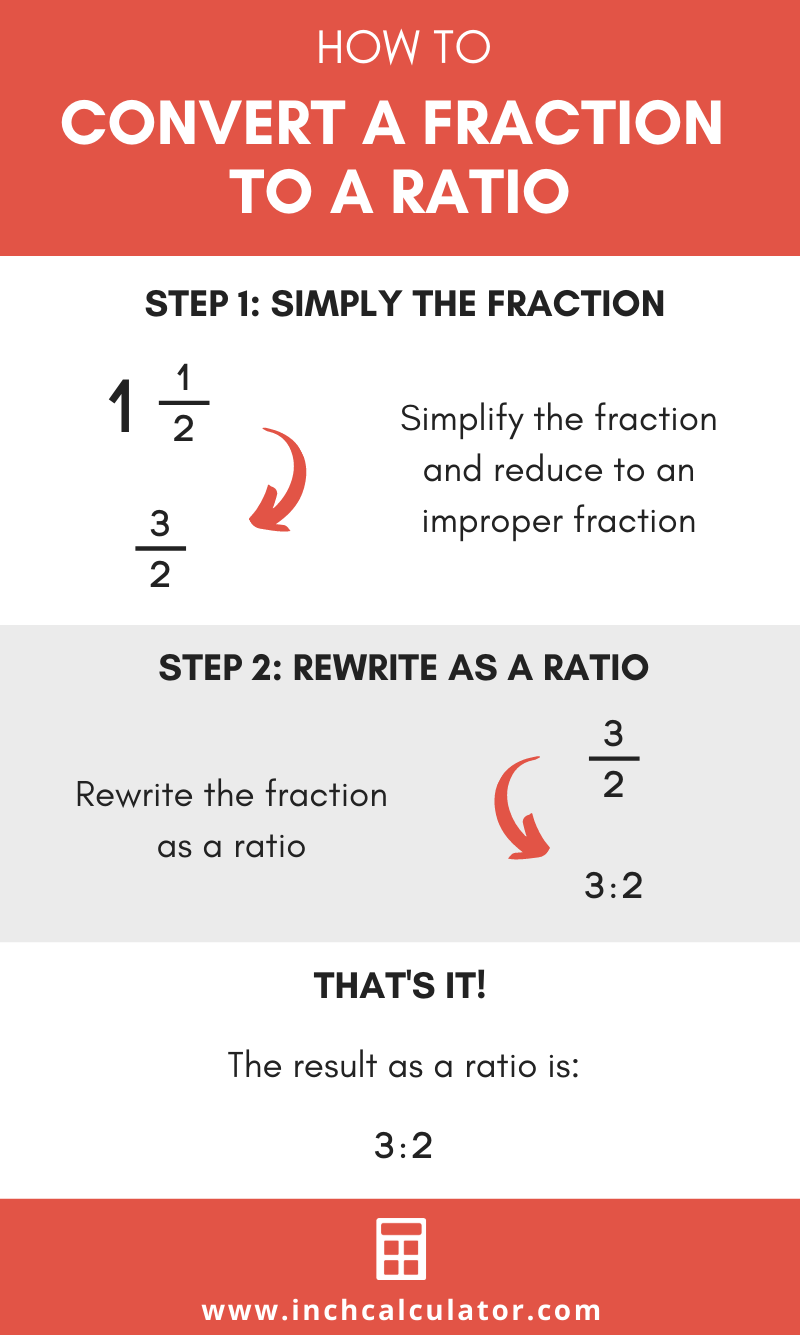# Fraction to Ratio Calculator

Convert a fraction to a ratio by entering the numerator and denominator below.

Fraction:

## Ratio:

3 : 4

### Steps to Convert a Fraction to Ratio

Step One: Reduce the fraction
6 / 8=3 / 4
Step Two: Rewrite as a ratio
3 / 4=3 : 4
Learn how we calculated this below

## How to Convert a Fraction to a Ratio

A fraction can be expressed as a ratio by following a few simple steps. The easiest way is to use the calculator above, which shows each step in the conversion.

Follow along to learn how to do it without a calculator.

### Step One: Simply and Convert to an Improper Fraction

First, simplify the fraction if it is not already reduced. If the fraction is a mixed number, then convert it to an improper fraction.

The result should be a reduced numerator and denominator only, with no whole number.

### Step Two: Rewrite as a Ratio

The second step is to rewrite the fraction as a ratio. A ratio is expressed as A:B, where A is the fraction numerator, and B is the fraction denominator.

For example, let’s convert 1 1 / 2 to a ratio.

Start by converting 1 1 / 2 to an improper fraction.
1 1 / 2 = 3 / 2

Then, rewrite 3 / 2 as a ratio.
3 / 2 = 3:2You might also be interested in our ratio to fraction calculator.